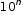# MA.8.NSO.1.4

Express numbers in scientific notation to represent and approximate very large or very small quantities. Determine how many times larger or smaller one number is compared to a second number.

### Examples

Roderick is comparing two numbers shown in scientific notation on his calculator. The first number was displayed as 2.3147E27 and the second number was displayed as 3.5982E-5. Roderick determines that the first number is about 10³² times bigger than the second number.
General Information
Subject Area: Mathematics (B.E.S.T.)
Strand: Number Sense and Operations
Date Adopted or Revised: 08/20
Status: State Board Approved

## Related Courses

This benchmark is part of these courses.
1205050: M/J Accelerated Mathematics Grade 7 (Specifically in versions: 2014 - 2015, 2015 - 2020, 2020 - 2022, 2022 and beyond (current))
1205070: M/J Grade 8 Pre-Algebra (Specifically in versions: 2014 - 2015, 2015 - 2022, 2022 and beyond (current))
1204000: M/J Foundational Skills in Mathematics 6-8 (Specifically in versions: 2014 - 2015, 2015 - 2022, 2022 and beyond (current))
7812030: Access M/J Grade 8 Pre-Algebra (Specifically in versions: 2014 - 2015, 2015 - 2018, 2018 - 2019, 2019 - 2022, 2022 and beyond (current))

## Related Access Points

Alternate version of this benchmark for students with significant cognitive disabilities.
MA.8.NSO.1.AP.4: Multiply a single-digit number by the power of 10 using a calculator. Identify whether the number in scientific notation represents a very large or very small quantity.

## Related Resources

Vetted resources educators can use to teach the concepts and skills in this benchmark.

## Formative Assessments

Estimating Extreme Values:

Students are asked to estimate an extremely large and an extremely small number by writing it in the form a x.

Type: Formative Assessment

Order Matters:

Students are given pairs of numbers written in the form of an integer times a power of 10 and are asked to compare the numbers in each pair using the inequality symbols.

Type: Formative Assessment

How Many Times?:

Students are given pairs of numbers written in exponential form and are asked to compare them multiplicatively.

Type: Formative Assessment

Compare Numbers:

Students are given pairs of numbers written in scientific notation and are asked to compare them multiplicatively.

Type: Formative Assessment

Scientific Calculator Display:

Students are given examples of calculator displays and asked to convert the notation in the display to both scientific notation and standard form.

Type: Formative Assessment

## Original Student Tutorial

Scientific Notation: Expressing Large Quantities:

Explore how to express large quantities using scientific notation in this interactive tutorial.

Type: Original Student Tutorial

## MFAS Formative Assessments

Compare Numbers:

Students are given pairs of numbers written in scientific notation and are asked to compare them multiplicatively.

Estimating Extreme Values:

Students are asked to estimate an extremely large and an extremely small number by writing it in the form a x.

How Many Times?:

Students are given pairs of numbers written in exponential form and are asked to compare them multiplicatively.

Order Matters:

Students are given pairs of numbers written in the form of an integer times a power of 10 and are asked to compare the numbers in each pair using the inequality symbols.

Scientific Calculator Display:

Students are given examples of calculator displays and asked to convert the notation in the display to both scientific notation and standard form.

## Original Student Tutorials Mathematics - Grades 6-8

Scientific Notation: Expressing Large Quantities:

Explore how to express large quantities using scientific notation in this interactive tutorial.

## Student Resources

Vetted resources students can use to learn the concepts and skills in this benchmark.

## Original Student Tutorial

Scientific Notation: Expressing Large Quantities:

Explore how to express large quantities using scientific notation in this interactive tutorial.

Type: Original Student Tutorial

## Parent Resources

Vetted resources caregivers can use to help students learn the concepts and skills in this benchmark.